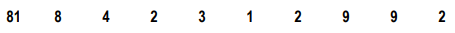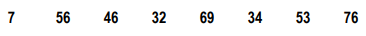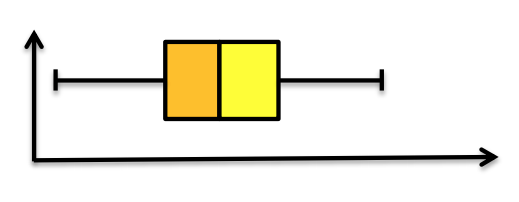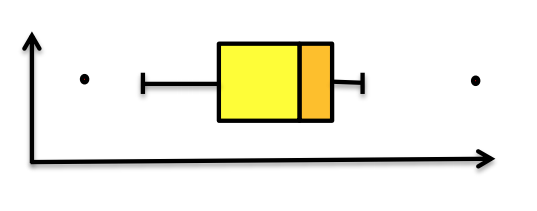Clutch Prep is now a part of Pearson
Ch.2: Measures of Center and SpreadWorksheetSee all chapters

# Boxplots (The Five Number Summary)

See all sections
Sections
Measures of Central Tendency
Measures of Spread: Standard Deviation
Measures of Spread: Interquartile Range
Boxplots (The Five Number Summary)

Concept #1: Determining The Five Number Summary and Drawing Boxplots

###### Expert Q&A

Ask unlimited questions and get expert help right away.

Concept #2: Determining The Five Number Summary and Drawing Boxplots: Intro

###### Expert Q&A

Ask unlimited questions and get expert help right away.

Practice: Determine the mean and median for the following data set:Practice: Determine the mean and median for the following data set:Practice: From the boxplot in Practice 2, what can be said about the data set in Practice 1?

Practice: What can be said about the data set that generated the boxplot below?Practice: What can be said about the data set that generated the boxplot below?###### Expert Q&A

Ask unlimited questions and get expert help right away.